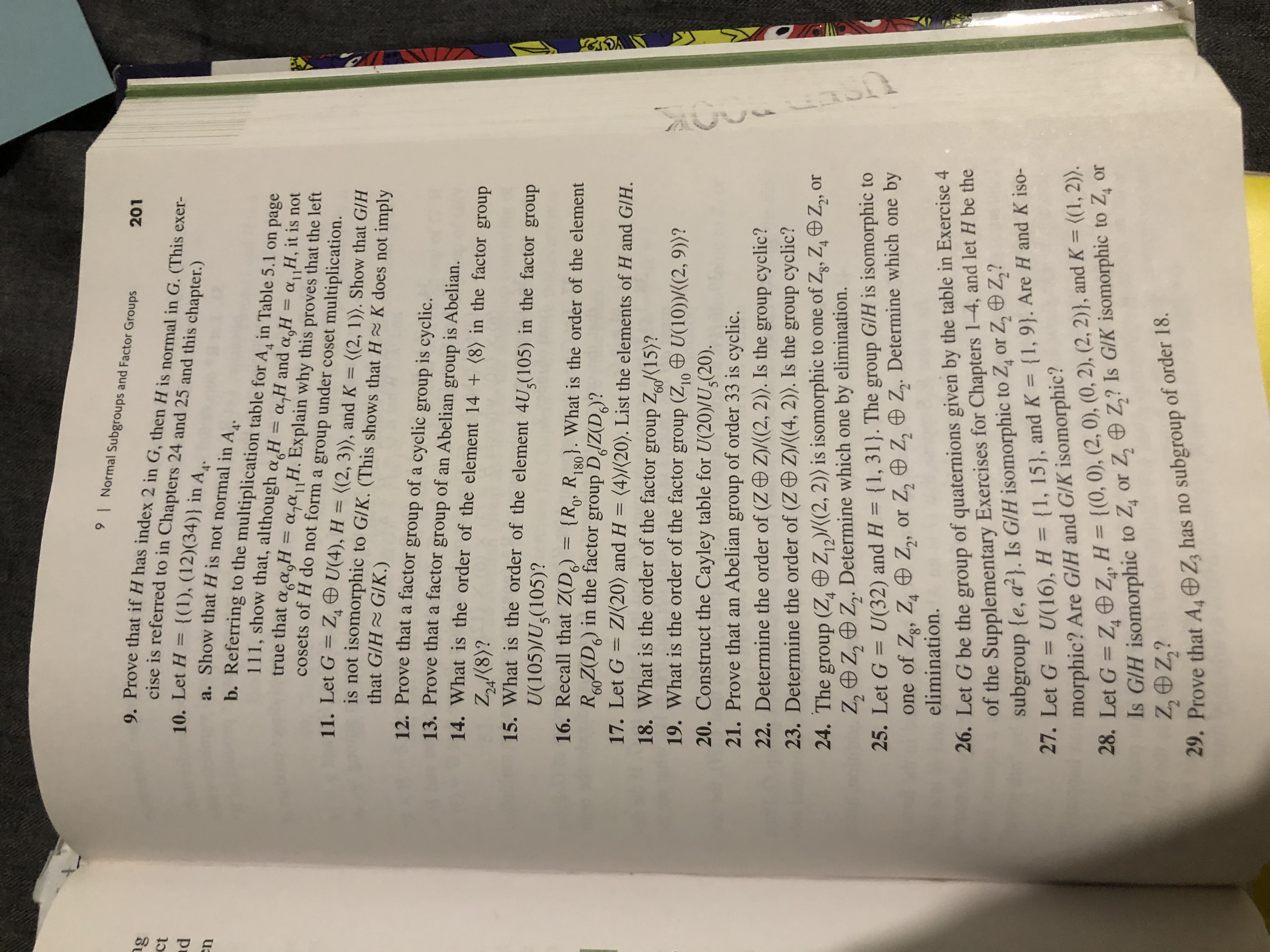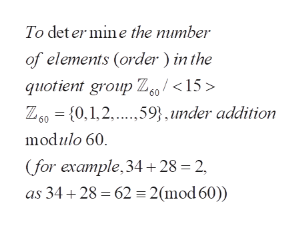# 9Normal Subgroups and Factor Groups2019. Prove that if H has index 2 in G, then H is normal in G. (This exer-cise is referred to in Chapters 24 and 25 and this chapter.)ggctd10. Let H {(1), (12)(34)} in A,.a. Show that H is not normal in A,.nb. Referring to the multiplication table for A, in Table 5.1 on page111, show that, although a H = a,H and a,H = a,,H, it is notthat agasH= a,a1cosets of H do not form a group under coset multiplication.H.Explain why this proves that the left11. Let G Z U(4), H = ((2, 3), and K =is not isomorphic to G/K. (This shows that H~ K does not implythat G/H GIK.)12. Prove that a factor group of a cyclic group is cyclic.13. Prove that a factor group((2, 1)). Show that G/HofAbelian group is Abelian.14. What is the order of the element 14 (8) in the factorangroupZn(8)?15. What is the order of the element 4U(105) in the factor groupU(105)/U,(105)?16. Recall that Z(D) =RZ(D) in the factor group DdZ(D?{Rp, R). What is the order of the element18060ZI20) and H = (4)/(20). List the elements of H and G/H.17. Let G18. What is the order of the factor group Z15)?19. What is the order of the factor group (Z U(10))/(2, 9))?20. Construct the Cayley table for U(20)/U(20).601021. Prove that an Abelian group of order 33 is cyclic.22. Determine the order of (Z D Z)((2, 2). Is the group cyclic?Z)/((4, 2). Is the group cyclic?23. Determine the order of (Z24. The group (Z, Z2)(2, 2)) is isomorphic to one of Zg, Z Z, orZ, Z, Z,. Determine which one by elimination.25. Let G U(32) and H (1, 31). The group GIH is isomorphic toone of Zg, Z Z, or Z, e Z, Z. Determine which one byelimination.426. Let G be the group of quaternions given by the table in Exercise 4of the Supplementary Exercises for Chapters 1-4, and let H be thesubgroup {e, a2}. Is G/H isomorphic to Z, or Z, Z,?27. Let G = U(16), H = {1, 15), and K {1, 9}. Are H and K iso-morphic? Are G/H and G/K isomorphic?28. Let G Z, Z, H= {(0, 0), (2, 0), (0, 2), (2, 2)}, and K= {(1, 2)).Is GIH isomorphic to Z, or Z, Z,? Is GIK isomorphic to Z, orZ,Oz?4429. Prove that AOZ has no subgroup of order 18Naull

Question
35 views

18help_outlineImage Transcriptionclose9 Normal Subgroups and Factor Groups 201 9. Prove that if H has index 2 in G, then H is normal in G. (This exer- cise is referred to in Chapters 24 and 25 and this chapter.) gg ct d 10. Let H {(1), (12)(34)} in A,. a. Show that H is not normal in A,. n b. Referring to the multiplication table for A, in Table 5.1 on page 111, show that, although a H = a,H and a,H = a,,H, it is not that agasH= a,a1 cosets of H do not form a group under coset multiplication. H.Explain why this proves that the left 11. Let G Z U(4), H = ((2, 3), and K = is not isomorphic to G/K. (This shows that H~ K does not imply that G/H GIK.) 12. Prove that a factor group of a cyclic group is cyclic. 13. Prove that a factor group ((2, 1)). Show that G/H of Abelian group is Abelian. 14. What is the order of the element 14 (8) in the factor an group Zn(8)? 15. What is the order of the element 4U(105) in the factor group U(105)/U,(105)? 16. Recall that Z(D) = RZ(D) in the factor group DdZ(D? {Rp, R). What is the order of the element 180 60 ZI20) and H = (4)/(20). List the elements of H and G/H. 17. Let G 18. What is the order of the factor group Z15)? 19. What is the order of the factor group (Z U(10))/(2, 9))? 20. Construct the Cayley table for U(20)/U(20). 60 10 21. Prove that an Abelian group of order 33 is cyclic. 22. Determine the order of (Z D Z)((2, 2). Is the group cyclic? Z)/((4, 2). Is the group cyclic? 23. Determine the order of (Z 24. The group (Z, Z2)(2, 2)) is isomorphic to one of Zg, Z Z, or Z, Z, Z,. Determine which one by elimination. 25. Let G U(32) and H (1, 31). The group GIH is isomorphic to one of Zg, Z Z, or Z, e Z, Z. Determine which one by elimination. 4 26. Let G be the group of quaternions given by the table in Exercise 4 of the Supplementary Exercises for Chapters 1-4, and let H be the subgroup {e, a2}. Is G/H isomorphic to Z, or Z, Z,? 27. Let G = U(16), H = {1, 15), and K {1, 9}. Are H and K iso- morphic? Are G/H and G/K isomorphic? 28. Let G Z, Z, H= {(0, 0), (2, 0), (0, 2), (2, 2)}, and K= {(1, 2)). Is GIH isomorphic to Z, or Z, Z,? Is GIK isomorphic to Z, or Z,Oz? 4 4 29. Prove that AOZ has no subgroup of order 18 N aull fullscreen
check_circle

Step 1

To determine the order of the given quotient group

Step 2

Describint the group Z(subscript)60help_outlineImage TranscriptioncloseTo det er mine the number of elements (order ) in the quotient group Z0/<15 > Z60 = {0,1,2....,59}, under addition modulo 60 (for example,34+28 2 as 34 28 62 2(mod 60)) fullscreen
Step 3

Describing the cyclic g...

### Want to see the full answer?

See Solution

#### Want to see this answer and more?

Solutions are written by subject experts who are available 24/7. Questions are typically answered within 1 hour.*

See Solution
*Response times may vary by subject and question.
Tagged in

### Math